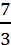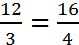# Ratios and Proportions

This section introduces the concepts of ratio and proportion. Chunks of the material in this section are excerpted from Ferdinand Hassler’s 1826 book, Elements of Arithmetic, Theoretical and Practical. The book was written for use in American schools and in private study.

In the notebook, Macdonald delineates two kinds of ratios, arithmetical and geometrical. Arithmetical ratios depend on addition and subtraction while geometrical ratios depend on multiplication and division. Macdonald follows this with a verbatim excerpt, with the exception of a copying error, from page 110 of Hassler:

“These two kinds of ratio take their mark of notation from the marks applied to the combinations or rules of arithmetic on which they depend; thus, the arithmetical ratio of 7 to 3 [is expressed by 7 – 3 and the geometrical ratio of 7 to 3] is expressed by 7:3 or.”

Macdonald left out the material in square brackets [ ]. Perhaps he was distracted or just not interested enough in the subject to pay attention to what he was doing.

This is followed by excerpts from pages 110 and 111 of Hassler that Macdonald’s teacher, George Baxter has slightly changed and edited. Macdonald writes:

“In the habitual mode of writing, therefore an arithmetical ratio expresses a difference between two quantities, and a geometrical ratio expresses the quotient arising from the division of two quantities. It is evident that two or more such ratios may be exactly equal to each other; such an equality of ratio is called a proportion or equations. Hence an arithmetical proportion may be expressed thus 7-3=12-8 and a geometrical proportion thus 12:3=16:4 or.

The first term of a ratio is call the antecedent, the second the consequent; the first and last terms of a proportion are called the extremes; the second and third the means.”

A minor change, for example, that Baxter made was to write, “It is evident” where Hassler wrote, “The simplest reflection leads to the idea:” A less minor change is that Baxter left out defining the index of a geometrical ratio, the number obtained when dividing two quantities. For example, 4 is the index of the ratios 12:3 and 16:4.